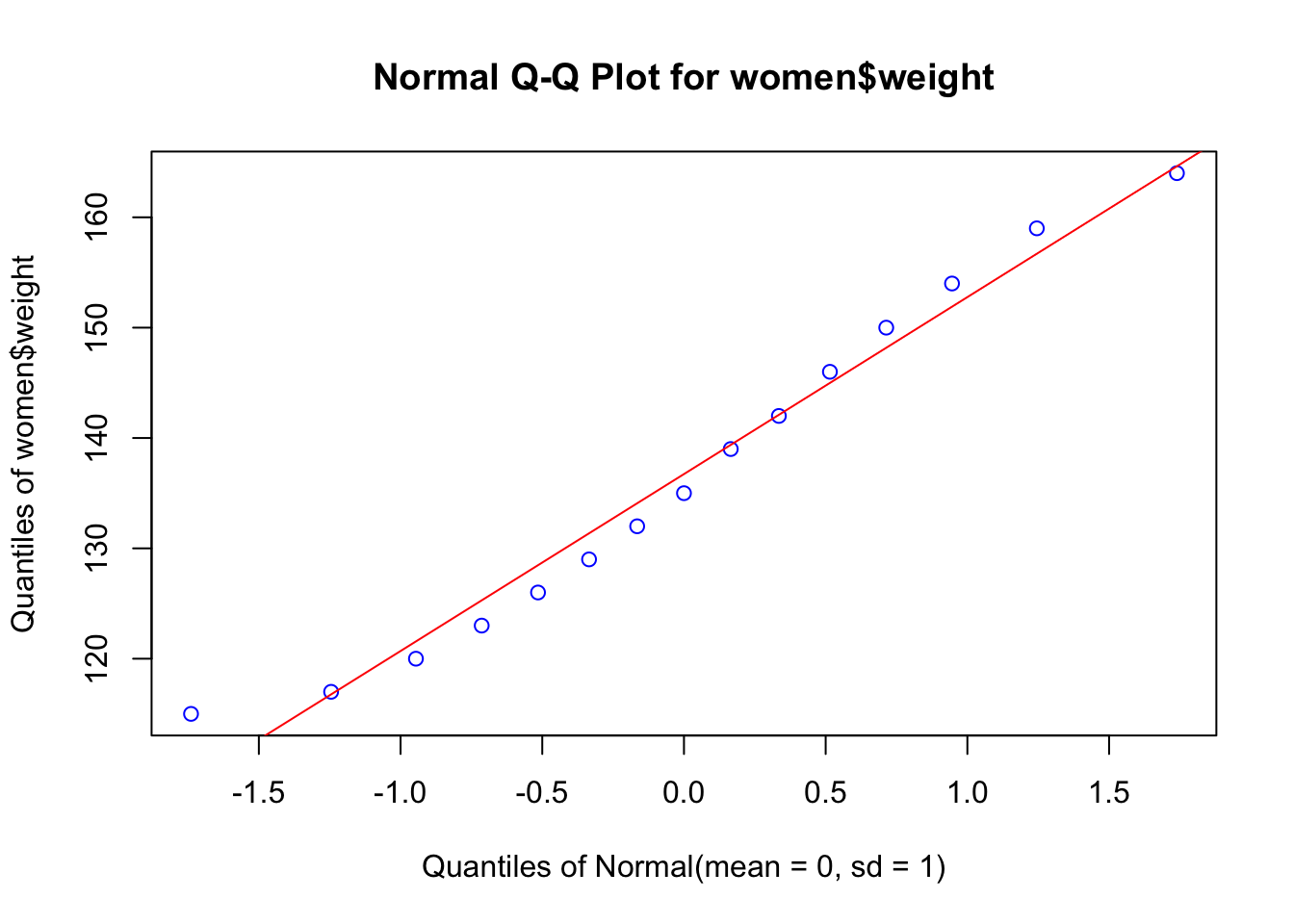## Statistical tests and graphs for the relationship between two variables

In this section we will consider the testing of statistical hypothesis for the relationship of two or more variables, and the graphs that will help in the interpretation of the results.

### Regression analysis

Regression analysis is a broad term for a set of methodologies used to predict a response variable (also called a dependent, criterion, or outcome variable) from one or more predictor variables (also called independent or explanatory variables). In general, regression analysis can be used to identify the explanatory variables that are related to a response variable, to describe the form of the relationships involved, and to provide an equation for predicting the response variable from the explanatory variables.

#### Simple linear regression

When the regression model contains one dependent variable and one independent variable, the approach is called simple linear regression.

The data set women in the base installation provides the height and weight for a set of 15 women ages 30 to 39. Suppose you want to predict weight from height. Having an equation for predicting weight from height can help you to identify overweight or underweight individuals.

But first, we have to prove that our data are close to a normal distribution, an assumption of the parametric methods, like linear regression. First we will build a Q-Q plot that will show how good is the agreement between the quantiles of the data (using z transformation) and the theoretical quantile of the corresponding normal distribution ($$\mu = 0, \sigma = 1$$)

library(EnvStats)
#Q-Q plot
women
qqPlot(women$weight, add.line = TRUE, points.col = "blue", line.col = "red")qqPlot(women$height, add.line = TRUE, points.col = "blue", line.col = "red")